Thermal analysis and calorimetry analysis

Ⅰ.thermal analysis

Thermal analysis is an important branch of instrumental analysis, which plays an irreplaceable role in the characterization of matter. After a long period of centuries, the heat has been aroused from the thermal analysis of minerals and metals. In recent decades, the polymer science and drug analysis have been full of vitality.

1. thermogravimetric analysis

Thermogravimetry Analysis (TG or TGA) is used to control the mass of a sample with temperature or time under the control of a certain temperature program (up/down/constant temperature) to obtain the weight loss ratio and weight loss temperature. Start point, peak value, end point…), and related information such as decomposition residual amount.
TG method is widely used in research and development, process optimization and quality monitoring of plastics, rubber, coatings, pharmaceuticals, catalysts, inorganic materials, metal materials and composite materials. The thermal stability and oxidative stability of the material under different atmospheres can be determined. The physical and chemical processes such as decomposition, adsorption, desorption, oxidation and reduction can be analyzed, including the use of TG test results for further apparent reaction kinetics. The material can be quantitatively calculated to determine the moisture, volatile components, and various additives and fillers.
The basic principle of the thermogravimetric analyzer is as follows: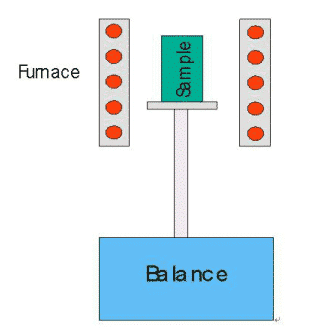The figure above shows the structure of the top-loading thermogravimetric analyzer. The furnace body is a heating body and operates under a certain temperature program. The furnace can be subjected to different dynamic atmospheres (such as N2, Ar, He and other protective atmospheres, O2, air and other oxidizing atmospheres and other special atmospheres, etc.), or The test was carried out under vacuum or static atmosphere. During the test, the high-precision balance connected to the lower part of the sample holder senses the current weight of the sample at any time, and transmits the data to the computer. The computer draws the sample weight versus temperature/time curve (TG curve). When the weight change of the sample (the reasons include decomposition, oxidation, reduction, adsorption and desorption, etc.), it will appear as a weight loss (or weight gain) step on the TG curve, so that the loss/weight gain process can be known. The temperature zone that occurred and quantify the loss/weight ratio. If a differential calculation is performed on the TG curve to obtain a thermogravimetric differential curve (DTG curve), more information such as the rate of change of weight can be obtained.
The typical thermogravimetric curve is shown below: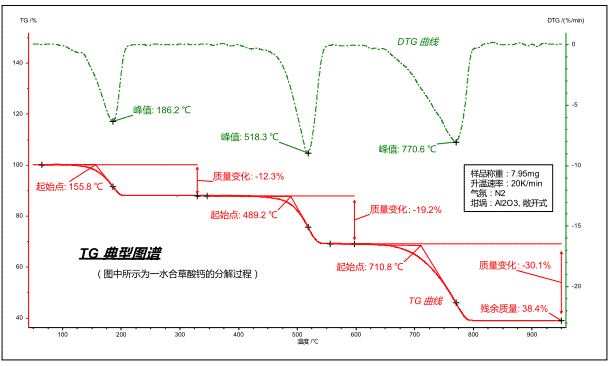The map can be converted in both temperature and time coordinates.
Red curve: The thermogravimetric (TG) curve characterizes the weight of a sample as a function of temperature/time during program temperature. The ordinate is the weight percent, which is the ratio of the weight of the sample to the initial weight at the current temperature/time.
Green curve: the thermogravimetric differential (DTG) curve (ie, the dm/dt curve, the curve of each point on the TG curve versus the time coordinate), which characterizes the rate of change of weight with temperature/time, and its peak point is characterized. The temperature/time point at which the weight change rate of each loss/weight gain step is the fastest.
For a loss/growth step, the following feature points are more commonly used:
The extrapolation starting point of the TG curve: the intersection point of the tangent line at the level before the TG step and the tangent point at the inflection point of the curve can be used as the reference temperature point at which the loss/weight gain process starts, and is mostly used to characterize the thermal stability of the material.
TG curve extrapolation termination point: the intersection point of the tangent line at the level after the TG step and the tangent point at the curve inflection point can be used as the reference temperature point at the end of the loss/weight gain process.
DTG curve peak: The temperature/time point at which the mass change rate is the largest, corresponding to the inflection point on the TG curve.
Mass change: Analyze the mass difference between any two points on the TG curve to represent the mass change of the sample caused by a weight loss (or weight gain) step.
Residual mass: The mass remaining in the sample at the end of the measurement.
In addition, in the software, the inflection point of the TG curve (equivalent to the DTG peak temperature), the DTG curve extrapolation starting point (closer to the true reaction initiation temperature), and the DTG curve extrapolation termination point (closer to Characteristic parameters such as the reaction end temperature in the true sense are marked.

2.calorimetric analysis

Calorimetry is a discipline that studies how to measure the changes in heat accompanying various processes. Accurate thermal property data can in principle be obtained by calorimetric experiments, which are carried out by calorimeters.
Differential thermal analysis (DTA) is a thermal analysis method that measures the temperature difference between a sample and a reference at a programmed temperature. Differential Scanning Calorimetry (DSC) is a thermal analysis method that measures the relationship between the power difference and temperature input to a sample and a reference under programmed temperature conditions. The physical meanings of the two methods are different. DTA can only test temperature characteristic points such as phase transition temperature. DSC can not only measure the phase change temperature point, but also measure the heat change during phase change. The exothermic peak and endothermic peak on the DTA curve have no definite physical meaning, while the exothermic peak and endothermic peak on the DSC curve represent heat release and heat absorption, respectively. Therefore, we use DSC as an example to analyze the calorimetric analysis.
Differential Scanning Calorimetry (DSC) is to observe the change of the heat flow power difference between the sample end and the reference end with temperature or time under the control of a certain temperature program (up/down/constant temperature). In this way, the thermal effect information of the sample during the temperature program, such as endothermic, exothermic, specific heat change, etc., is calculated, and the heat absorption (heat enthalpy) and characteristic temperature (starting point, peak value, end point…) of the thermal effect are calculated.
The DSC method is widely used in various fields such as plastics, rubber, fibers, coatings, adhesives, medicines, foods, biological organisms, inorganic materials, metal materials and composite materials. It can study the melting and crystallization process of materials, glass transition, Phase transition, liquid crystal transition, solidification, oxidation stability, reaction temperature and reaction enthalpy, the specific heat and purity of the substance are measured, the compatibility of each component of the mixture is studied, and the crystallinity and reaction kinetic parameters are calculated.
The basic principle of the heat flow differential scanning calorimeter is as follows: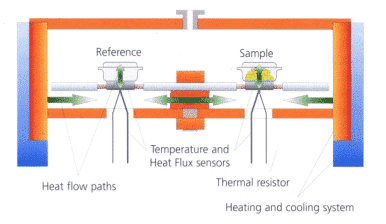As shown in the figure above, the sample is packed with a sample and placed on the sensor disk together with a reference crucible (usually empty). The two are kept thermally symmetrical and in a uniform furnace according to a certain temperature program (linear heating) , cooling, constant temperature, and combinations thereof) were tested and a pair of thermocouples (reference thermocouple, sample thermocouple) were used to continuously measure the temperature difference between the two. Since the furnace body to sample/reference heating process satisfies the Fourier heat conduction equation, the heating heat flow difference at both ends is proportional to the temperature difference signal, so the original temperature difference signal can be converted into a heat flow difference signal by heat flow correction, and the time/temperature is Continuous mapping to obtain a DSC map.
The thermal effect of the sample causes a heat flow imbalance between the reference and the sample. Due to the presence of thermal resistance, the temperature difference between the reference and the sample () is proportional to the heat flow difference. The time will be integrated to get the heat: (temperature, thermal resistance, material properties…)
Due to the thermal symmetry of the two enthalpies, the signal difference between the reference end and the sample end is close to zero in the absence of thermal effects in the sample. An approximate horizontal line is obtained on the map, called the “baseline”. Of course, it is impossible for any actual instrument to achieve perfect thermal symmetry. In addition, the difference in heat capacity between the sample end and the reference end is usually not completely horizontal, and there is a certain undulation. This volt is usually called “baseline drift”.
When the sample has a thermal effect, a certain temperature difference/heat flow signal difference is generated between the sample end and the reference end. By continuously plotting the signal difference versus time/temperature, a map similar to the following can be obtained: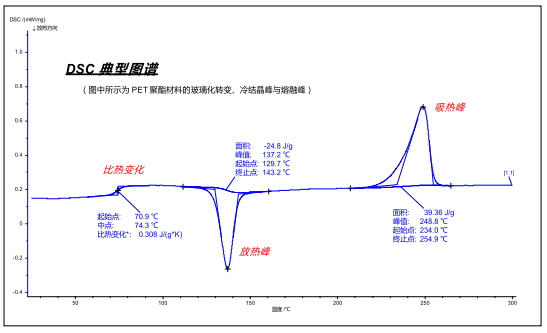According to the DIN standard and thermodynamic regulations, the upward (positive value) shown in the figure is the endothermic peak of the sample (the typical endothermic effect is melting, decomposition, desorption, etc.), and the downward (negative value) is the exothermic peak ( The typical exothermic effect is crystallization, oxidation, solidification, etc., and the specific heat change is reflected in the change of the baseline height, that is, the step-like inflection on the curve (the typical specific heat change effect is glass transition, ferromagnetic transition, etc.) ).
The map can be converted in both temperature and time coordinates.
For the absorption/exothermic peak, the starting point, peak value, end point and peak area can be analyzed more commonly. some of:
Starting point: The point at which the baseline before the peak is tangent to the tangent at the inflection point to the left of the peak, often used to characterize the temperature (time) at which a thermal effect (physical or chemical reaction) begins to occur.
Peak: The temperature (time) point at which the absorption/exothermic effect is greatest.
Termination point: The point at which the baseline after the peak is tangent to the tangent to the right of the peak, which corresponds to the starting point and is often used to characterize the temperature (time) at which a thermal effect (physical or chemical reaction) ends.
Area: The area obtained by integrating the absorption/exothermic peaks, in J/g, to characterize the amount of heat absorbed/discharged by a unit weight of a sample during a physical/chemical process.
In addition, characteristic parameters such as height, width, and area integral curve of the absorption/exothermic peak can be indicated in the software. For the specific heat change process, parameters such as the starting point, the midpoint, the end point, the inflection point, and the specific heat change value can be analyzed.

Ⅱ. thermal analysis equipment

1. thermogravimetric analyzer

The modern TG instrument has a complicated structure. In addition to the basic heating furnace and high-precision balance, there are electronic control parts, software, and a series of auxiliary equipment. The structure of the Netzsch TG209F3 is shown in the figure below:
The protective gas and the purge gas can be seen in the figure. The protective gas is usually inert to the N2. It is passed into the furnace through the Weighing chamber and the joint connection area, so that the balance can be placed. A stable and dry working environment that prevents moisture, hot air convection, and sample decomposition of pollutants from affecting the balance. The instrument allows two different purge gas types (purge1, purge2) to be connected at the same time and automatically switched or mixed during the measurement as needed. A common connection is one in which N2 is connected as an inert purge atmosphere for conventional applications; the other is connected to air as an oxidizing atmosphere. In terms of gas control accessories, it can be equipped with a conventional rotameter, solenoid valve, or a mass flow meter (MFC) with higher precision and automation.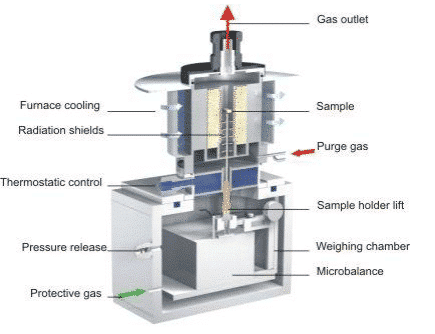The gas outlet is located at the top of the instrument and can be used to discharge carrier gas and gaseous products to the atmosphere. It can also be connected to FTIR, QMS, GC-MS and other systems using a heated transfer line to deliver product gases to these instruments. Component detection. The top loading structure of the instrument and the natural smooth gas path design make the carrier gas flow rate small, the product gas concentration high, and the signal hysteresis small, which is very beneficial for the combination with these systems for the effective analysis of the evolved gas components.
The instrument is equipped with a thermostatic control to isolate the furnace from the two parts of the balance, which can effectively prevent heat from being transferred to the balance module when the furnace is at a high temperature. In addition, the bottom-up continuous purge of the shielding gas prevents the heat transfer caused by the convection of the hot air, and the radiation shields around the sample holder isolate the heat radiation factors in the high temperature environment. The measures ensure that the high-precision balance is in a stable temperature environment and is not interfered by the high temperature zone, ensuring the stability of the thermogravimetric signal.

2. differential scanning calorimeter

Modern DSC instruments are more complex in structure, in addition to the basic heating furnace and sensors, as well as electronic control parts, software, and a range of auxiliary equipment. The diagram below shows the structure of the Netzsch DSC204F1: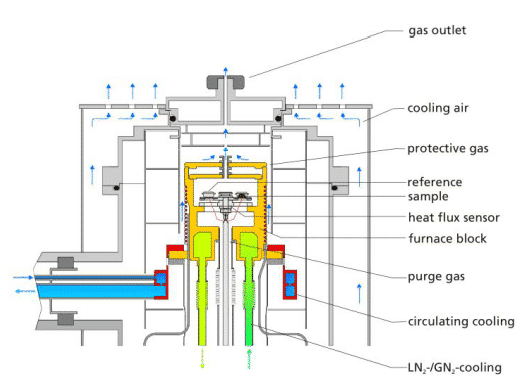The protective gas and the purge gas can be seen in the figure. The protective gas is usually passed through the periphery of the furnace using inert N2, which can protect the heating body, prolong the service life, and prevent the furnace body. The effect of frosting on the periphery at low temperatures. The instrument allows two different purge gas types to be connected simultaneously and automatically switched or mixed during the measurement as needed. Conventional connection is one in which N2 is connected as an inert purge atmosphere for conventional applications; the other is connected to air or O2 for use as an oxidizing atmosphere. In terms of gas control accessories, it can be equipped with a conventional rotameter, solenoid valve, or a mass flow meter (MFC) with higher precision and automation.
The instrument can be connected to three different types of cooling equipment. One is liquid nitrogen system LN2/GN2 cooling), one is circulating cooling or intracooler, and the other is cooling air. These three cooling methods each have different characteristics and suitable applications. Compressed air is relatively simple, the minimum cooling temperature is normal temperature, suitable for occasions that do not require low temperature applications (such as plastics, thermosetting resin industry, etc.), and is often used as automatic cooling after the end of measurement, so that the furnace body is cooled to normal temperature, easy Adding the next sample; liquid nitrogen system has the advantage of faster cooling and lowering to a lower temperature (-180 °C) than mechanical refrigeration. The disadvantage is that liquid nitrogen itself is a consumable. Need to add, there are factors of consumables cost; while mechanical refrigeration is inferior to liquid nitrogen in cooling rate and limit temperature, but the following basically no consumable factors can be used all the time, which is its advantage.

Ⅲ.the experimental factors affecting thermal analysis and measurement

1. Effect of heating rate on the results of thermal analysis experiments

The rate of temperature rise has a significant effect on the results of the thermal analysis experiment. In general, it can be summarized as follows.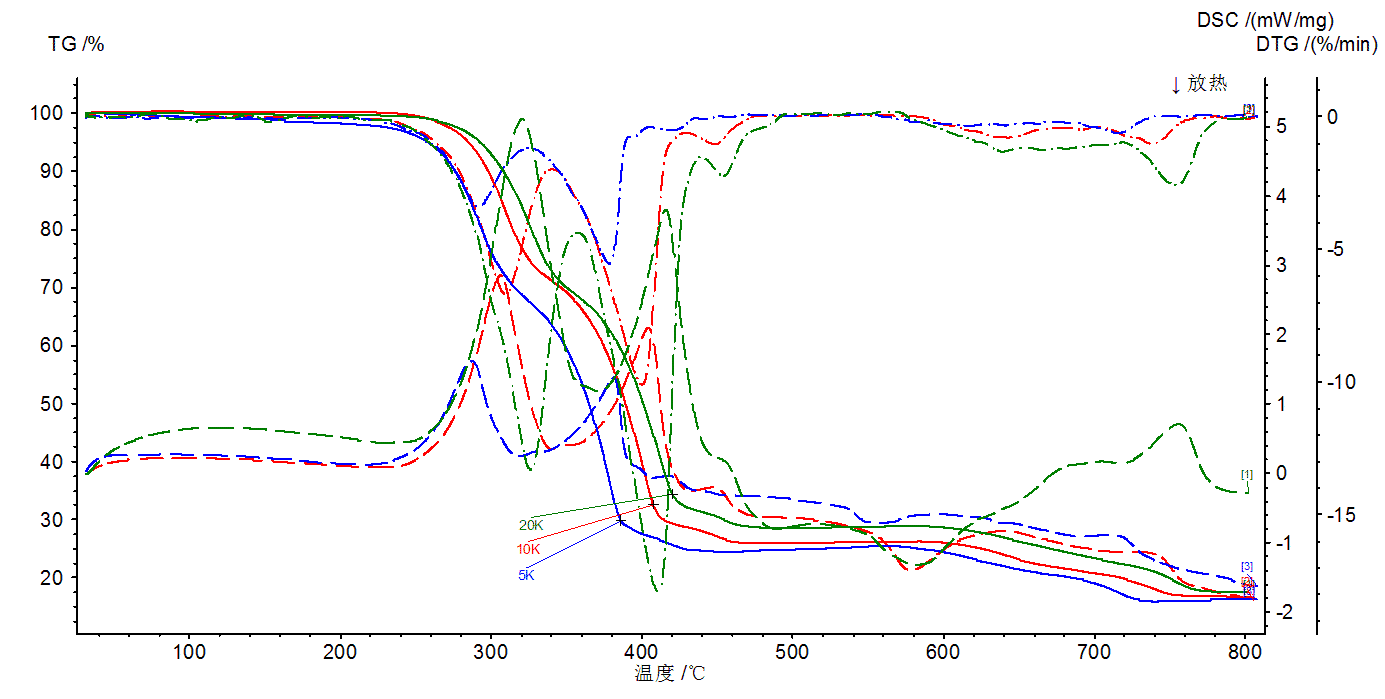(1) For a certain reaction of the sample represented by the TG, DSC curve, the increase in the temperature increase rate is usually such that the initial temperature of the reaction Ti, the peak temperature Tp, and the termination temperature Tf are increased. Rapid temperature rise, so that the reaction has not yet been able to proceed, it enters a higher temperature, the assembly reaction lags (pictured above).
(2) Rapid temperature rise is to push the reaction to a higher speed in a high temperature region, that is, not only the peak temperature Tp of the DSC curve is increased, but also the peak amplitude is narrowed and peaked (as shown in the above figure).

2. Effect of sample dosage and particle size on thermal analysis experiments

A small amount of sample is beneficial to the diffusion of the gas product and the internal temperature of the sample, reducing the temperature gradient and reducing the deviation of the sample temperature from the linear temperature rise of the environment, which is caused by the absorption and heat release effects of the sample. Experiments have shown that the peak area is still related to the particle size of the sample. The smaller the particle, the larger the area of the exothermic peak of the DSC curve. In addition, there is a gap between the piled loose sample particles, which makes the sample thermally deteriorated, and the smaller the particles, the closer the pile can be piled up and the heat conduction is good. Regardless of the particle size of the sample, the penguin density is not very easy to repeat and will also affect the TG curve topography.

3. Influence of atmosphere on the results of thermal analysis experiments

For the reaction to form a gaseous product, if the gas product is not removed in time, or the partial pressure of the gaseous product in the atmosphere is increased by other means, the reaction is moved to a high temperature. The thermal conductivity of the atmosphere is good, which is beneficial to provide more heat to the system and increase the rate of decomposition reaction. The relationship between the thermal conductivity of three inert gases of argon, nitrogen and helium and temperature is increasing in sequence.
The figure below shows the decomposition test of dolomite. The decomposition process consists of the following two steps:
MgCO3 → MgO + CO2 ↑
CaCO3 → CaO + CO2 ↑
Under the condition of conventional N2 purging, the temperature of the two decomposition steps is similar, and the separation effect is not good. It is difficult to accurately calculate the content of the two components of MgCO3 and CaCO3. Therefore, CO2 was used as a purge atmosphere in this example. Since both weight-loss steps generate CO2, using CO2 as the purge atmosphere will affect the chemical balance and cause the reaction to “delay” (the weight loss ratio is not affected). Since the “delay degree” of the two-step decomposition is not the same, the delay of the second stage weight loss (CaCO3 decomposition) is more significant. In this way, the effect of step separation is effectively achieved, and the mass ratio of MgCO3 in the sample can be accurately calculated to be 44.0% (MgCO3/CO2=1.91), and the mass ratio of CaCO3 is 55.3% (CaCO3/CO2=2.27).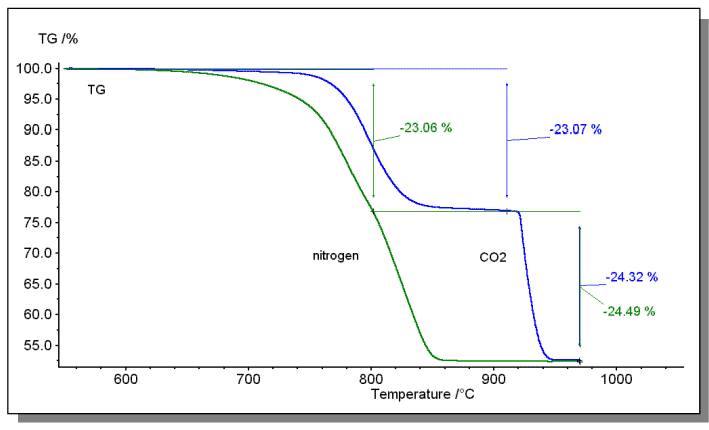4. Influence of buoyancy, convection and turbulence on TG curve

The gas phase density of the medium space in the sample holder decreases with the increase of temperature, and thus the buoyancy decreases, which is expressed as apparent weight gain. For the sample container, the upward flowing air causes apparent weight loss, and the two air turbulence causes weight gain, which is related to the size and shape of the crucible, which can be adjusted by means of the air outlet above the sample container, but the TG curve is made. It is difficult to have no apparent mass change over the entire temperature range.

5. the impact of the tightness of the sample on the experimental results

The degree of tightness of the sample loaded in the crucible affects the diffusion of the pyrolysis gas product into the surrounding medium air and the contact of the sample with the atmosphere. For example, the second step of calcium oxalate monohydrate CaC2O4·H2O loses the reaction of carbon monoxide CO: CaC2O4·H2O → CaCO3 + CO ↑
When the medium is air, if the sample is loose and has sufficient oxidizing atmosphere, the DSC curve has an exothermic effect (peak temperature 511 °C), which is the oxidation of CO: 2CO + O2 → 2CO2, if the sample is relatively compact, it is in the absence In the oxygen state, the DSC curve has an endothermic effect. See below.
loose (1) and more full (2)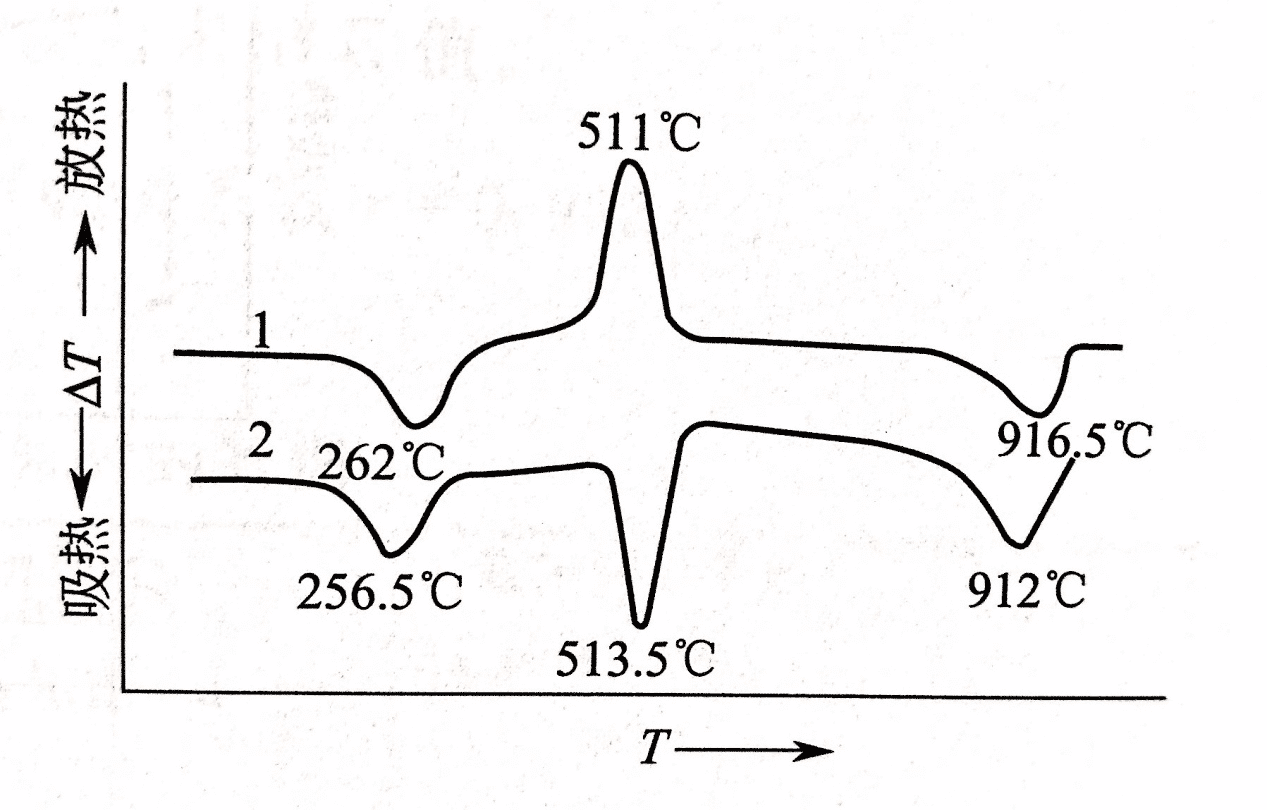Ⅳ.the measurement of various transformations by thermal analysis technology

1. Measurement of glass transition

For amorphous solids, glass transition occurs during heating, from amorphous solid to flow dynamics (highly elastic for polymeric materials). In this process, along with the change of specific heat, it is reflected in the DSC curve as a step toward the heat absorption direction.
Inflection. From this analysis, the glass transition temperature of the material can be obtained.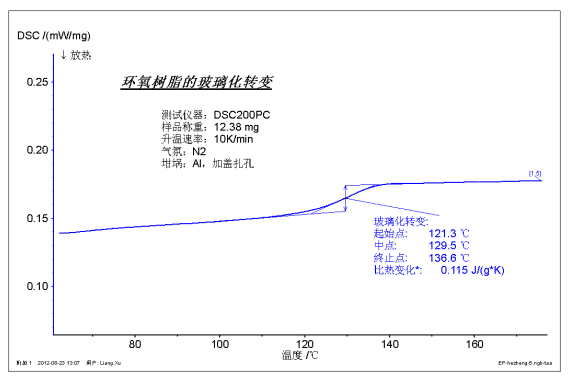The figure above shows the glass transition test of an epoxy resin sample. According to international standards, the glass transition generally takes the midpoint, which is 129.5 °C. The specific heat change roughly characterizes the severity of the transition.

2. Measurement of crystallization and melting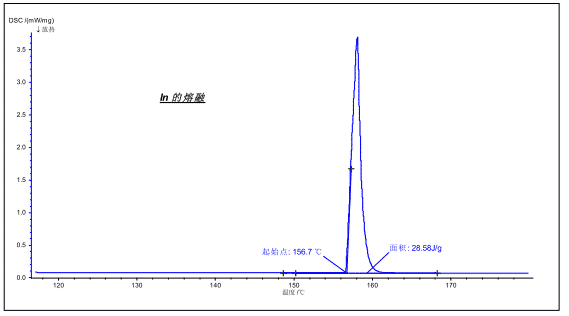The melting of the crystal is a first-order phase transition accompanied by an endothermic effect during the melting process. Using DSC, the endothermic effect can be measured to obtain information such as melting point, melting enthalpy, and the like.
The figure above shows the melting of the metal In. The melting point is 156.7 ° C (theoretical 156.6 ° C), the enthalpy is 28.58 J / g (theoretical value 28.6 J / g).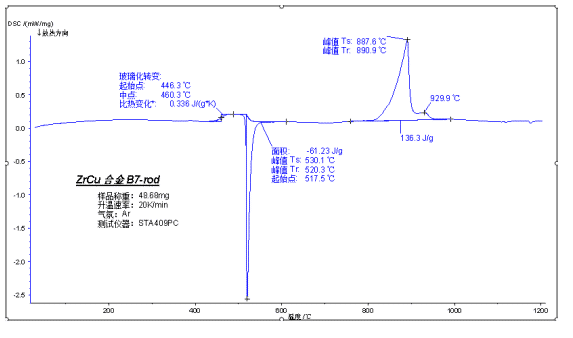The above figure shows the glass transition, cold crystallization and melting test of amorphous alloy during heating. Amorphous alloys have a high degree of amorphous phase due to insufficient crystallization at room temperature, so there is a significant glass transition during heating. A cold crystallization peak then appears, and the final melting peak contains the simultaneous melting of the crystal at room temperature and the added crystal portion of the cold crystallization process.

V. Typical analysis of thermal analysis

1. thermal stability

Using the thermogravimetric analyzer, by analyzing the initial stage of the decomposition process, it is easy to understand the thermal stability of the material and obtain information on the upper limit of the use temperature.
For the annotation of the temperature that can represent the thermal stability, the traditional external starting point method can be used (the TG step or the DTG peak can be used as the external starting point), but the temperature is subject to the analysis boundary condition (take the range of the tangent) Impact, sometimes not stable enough. In the industrial field and quality control occasions, more than 1%, 2%, 5% of the weight loss is used to characterize the thermal stability of the product, and the calculation result is more accurate and reliable.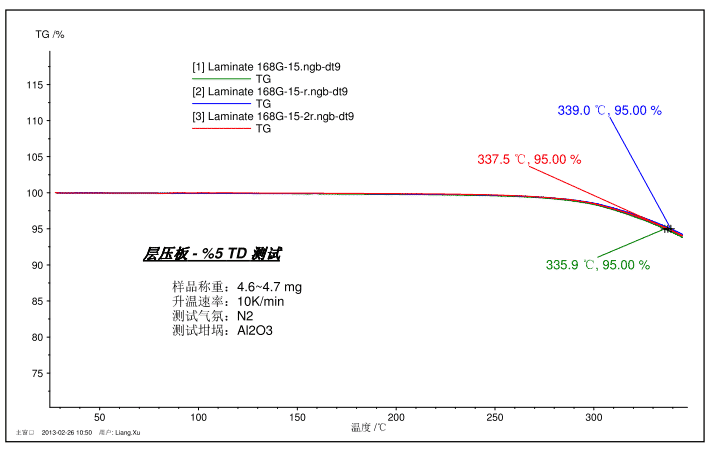The figure above shows a test spectrum of 5% TD (5% weight loss) of a laminate sample as a PCB material. The sample was tested three times in total, and the reproducibility was good, and the 5% TD was in the range of 337.5 ± 1.5 °C.

2. Pyrolysi process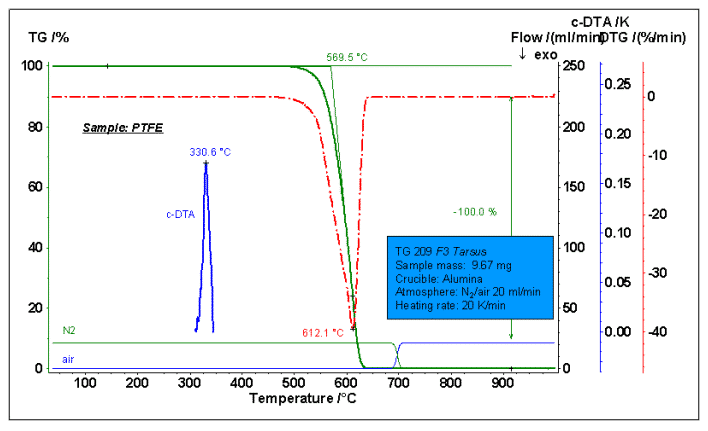The figure above shows the thermal degradation process test of polytetrafluoroethylene PTFE. The N2 atmosphere was used before 700 °C, and switched to air after 700 °C. PTFE is a high temperature resistant material, the initial decomposition temperature is as high as 500 ° C or above (the TG outer cutting starting point is 569.5 ° C in the figure), and the maximum weight loss rate point (DTG peak temperature) is 612.1 ° C. The sample was 100% completely weight loss under an inert atmosphere and no carbon residue was formed. This can be verified by switching from the graph to air without further weight loss. The c-DTA curve additionally gives a melting peak of PTFE at a temperature of 330.6 °C.

3. component analysis

Using a thermogravimetric analyzer, the internal component ratio of many materials can be calculated based on the multi-stage weight loss measurement results by using a suitable heating rate and measuring atmosphere, and rationally arranging switching between different atmospheres.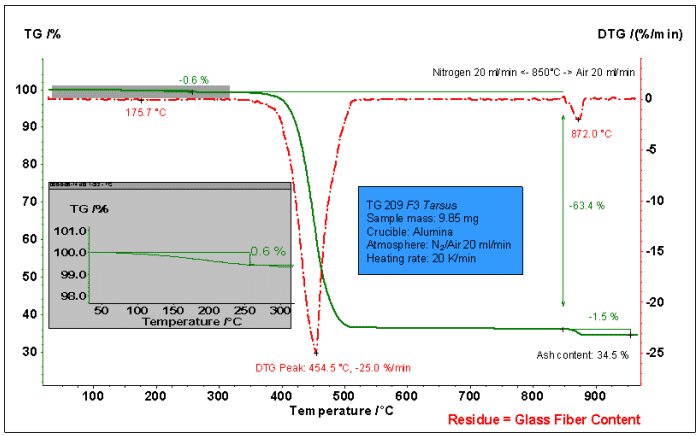The figure above shows the weight loss process analysis of glass fiber reinforced PA66. Use N2 before 850 °C, switch to air after 850 °C. It can be seen from the figure that the weight loss is divided into the following stages:
1. A small amount of weight loss before 1.300 °C: loss of weight 0.6%. May be the moisture adsorbed in the material and some organic volatiles.
2. 300~850°C: The main weight loss step, the weight loss is 63.4%. Decomposition of PA66.
3. After switching to air at 850 ° C: the weight loss is 1.5%, which corresponds to the loss of heat of carbon (PA66 decomposition product).
Residual quality: 34.5%. It should be a glass fiber component that does not decompose or oxidize.
From the above analysis, the ratio of PA66 in the sample can be calculated to be 64.9% (63.4+1.5). The proportion of glass fiber is 34.5%. The remaining moisture/volatile fraction was 0.6%.

4. volatile sublimation

Using a thermogravimetric analyzer, the volatilization process of a generation of samples (such as lubricating oil) can be tested and its stability characterized.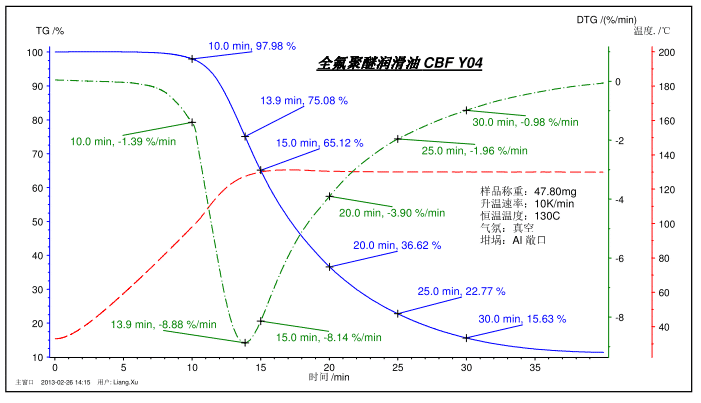The figure above shows the volatilization process test of perfluoropolyether lubricants. The temperature program was ramped from room temperature to 130 ° C and kept at a constant temperature. The figure shows the mass percentage at 10, 15, 20, 25, 30 min, and the fastest loss of focus at 13.9 min, and the corresponding DTG weight loss rate. Similarly, TG can also measure the volatilization (sublimation) process of certain solid samples, such as camphor, to characterize their storage stability.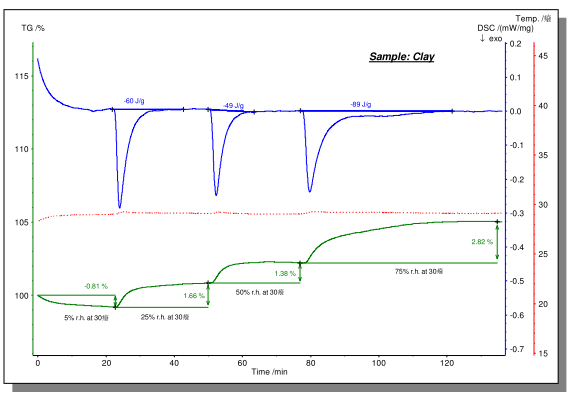The above figure shows the dehydration and water absorption process of the clay tested on the STA instrument under different humidity atmospheres. The test was carried out at a constant temperature of about 30 ° C using a humidity generator to create a purge atmosphere of a specific humidity. It can be seen that under a drier purge atmosphere of 5% relative humidity, the sample exhibited a dehydration process with a weight loss of 0.81%. When the atmosphere was switched to 25% relative humidity, the sample exhibited water absorption with a weight gain of 1.66%. Subsequent to 50% and 75% relative humidity, the samples all absorbed water, and the weight gain was 1.38% and 2.82%, respectively. At the same time, on the blue DSC curve, the exothermic effect and enthalpy of the water absorption process can be observed.

6. Effect of cooling rate on crystallinity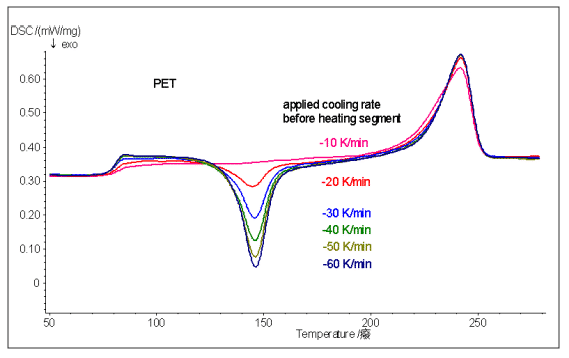The above figure compares the results obtained by another second temperature rise after another PET sample is cooled from the molten state to a normal temperature using a different cooling rate. It can be seen that the faster the cooling rate is, the less the crystallization of the sample is, and the larger the cold crystallization peak area obtained by the second heating, the lower the crystallinity.
Different crystallinity will affect the material’s mechanical properties (flexibility, ductility, impact resistance, etc.), optical properties, solvent resistance, and processability. Therefore, in the production process of thermoplastics, crystallinity is also an important indicator for detection and control.

7. Oxidation stability

The oxidative stability of the material can be tested using DSC. Specific test methods include the OIT method, and the dynamic temperature oxidation method.
Oxidation induction period (OIT) is a standard test method for the plastics industry. The constant temperature is generally 200 ° C, but the appropriate up / down adjustment can be made according to the length of the oxidation time. According to the difference of oxidation induction time (OIT) of different batches of samples, the difference of anti-oxidation performance of materials and the anti-oxidation effect of different anti-oxidation additives can be compared, and can be used indirectly to identify the difference of anti-aging properties of materials. Relevant measurement standards: DIN EN 728, ISO/TR 10837, ASTM D 3895.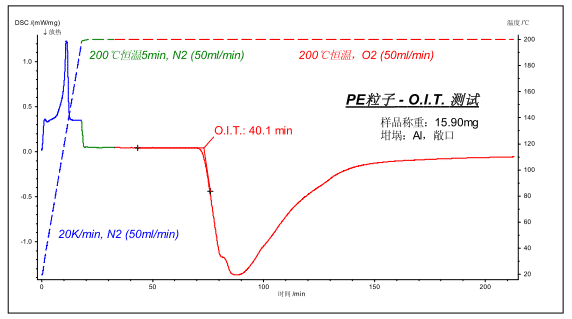The above picture shows the polyethylene plastic particle OIT test in accordance with the national standard method. The sample was weighed to about 15 mg, placed in an open Al crucible, and warmed to 200 ° C under 50 ml/min N 2 protection, and switched to O 2 after 5 min. The measured oxidation induction period (the time difference from the initial switching to O2 to the extrapolation initiation point of the oxidative exothermic peak) was 40.1 min.

8. curing test

DSC can measure the curing process of thermosetting resins (such as epoxy resins, phenolic resins, etc.), as well as coatings, adhesives, and the like.
The following figure shows the temperature rise curing test of glass fiber reinforced epoxy resin (GFEP) prepreg. The uncured prepreg has a low glass transition temperature (101.5 °C) and solidifies during the heating process. It shows a large exothermic peak on the DSC curve (136.4, 158.9 °C double peak in the figure, curing enthalpy 43.10) J/g); after cooling for a second temperature rise, since the resin has solidified, the glass transition temperature is raised to 142.4 ° C, and the curing exothermic peak no longer appears.
Note: For epoxy resins, the glass transition temperature is close to the linearity of the degree of cure. The higher the degree of curing, the more complete the internal crosslinking of the material, the lower the mobility of the segment, and the higher the glass transition temperature.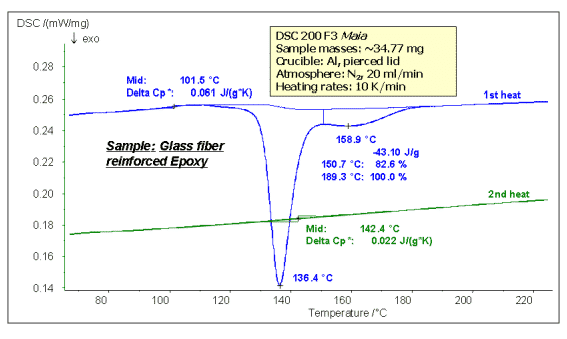9. phase change test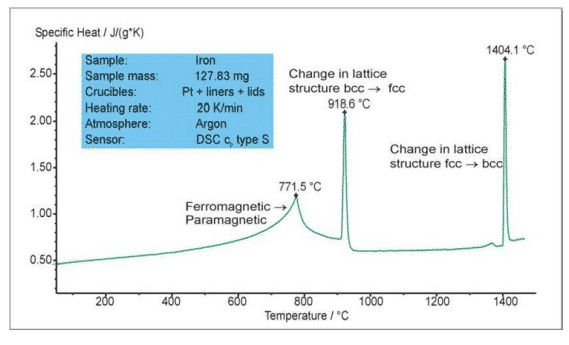The figure above shows the phase change test of iron during the heating process. The endothermic peak at 771.5 °C is the Curie point transition and the material is converted from ferromagnet to paramagnetic. The endothermic peak at 918.6 ° C and 1404.1 ° C is the transition between the two lattice structures (bcc body center – fcc face center). The Netzsch SC404/STA449 features a high-vacuum hermetic structure and a fully automated vacuum system with a unique OTS oxygen adsorption system to ensure that samples are measured in a pure inert atmosphere to avoid oxidation at elevated temperatures.

10. polymorph

Polymorphism refers to the phenomenon that a substance can exist in two or more different crystal structures. Various crystal forms have different physical and chemical properties and can be converted into each other under certain conditions.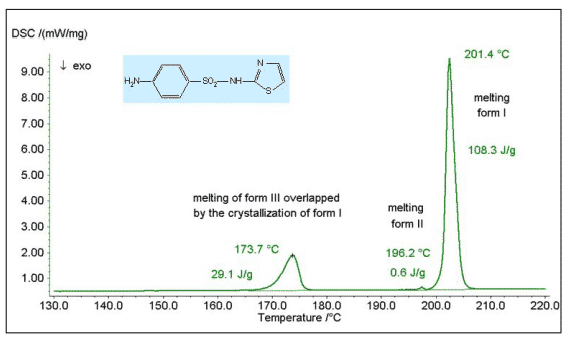The figure above shows the DSC measurement of the drug Sulfathiazole. The endothermic peak at 173.7 ° C in the figure is the melting of Form III, which is then converted to Form I. The small endothermic peak at 196.2 ° C is the melting of Form II, and the endothermic peak at 201.4 ° C is the melting of Form I.

11. specific heat test

Test principle
According to the definition of thermal physics, the specific heat capacity c (the constant thermal specific heat capacity Cp involved in the general thermal analysis) is the energy required to increase the unit temperature per unit mass of the sample at a certain temperature. Namely: Cp = Q / ( m * △ T ), unit J / g * K
Change this equation slightly:
Q = Cp * m * △T
Then differentiate the time, take the endothermic power of the sample during the heating process q = dQ / dt, the heating rate HR = dT / dt, that is: q = Cp * m * HR
Using the heat flow type DSC, the endothermic power q of the unknown specific heat sample sam and the known specific heat standard sample std at a certain temperature are respectively measured at the same heating rate in a dynamic heating rate, and it is obtained:
Qsam = KT * ( DSCsam – DSCbsl ) = Cpsam * msam * HR
Qstd = KT * ( DSCstd – DSCbsl ) = Cpstd * mstd * HR
KT is the sensitivity coefficient of the heat flow sensor, through which the DSC original signal (unit uV) at a certain temperature can be converted into a heat flow signal (unit mW). DSCbsl is a baseline that is measured using a pair of blanks and is deducted when measuring the heat flow of the sample and the standard.
Divide the above two equations, and KT and HR are divided by each other to obtain:
(DSCsam – DSCbsl) / (DSCstd – DSCbsl) =
(Cpsam * msam ) / ( Cpstd * mstd)
A slight change, that is, the constant pressure specific heat capacity of the sample at a certain temperature:
Cpsam = Cpstd × [(DSCsam – DSCbsl) / msam] / [(DSCstd – DSCbsl) / mstd] = Cpstd × DSCsam,rel,sub/ DSCstd,rel,sub
Where DSCxxx, rel, sub represents the DSC signal after the baseline or reference is subtracted from the baseline in relative coordinates, in μV/mg.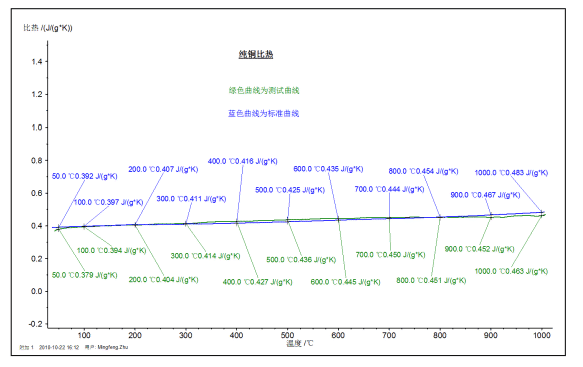The figure above shows the specific heat value (green curve) of a pure copper sample measured at high temperature DSC in the range of RT~1000 °C, and the comparison with the literature value (blue curve).22 July, 2020

عمل رائع جداً .